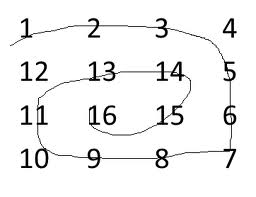# Print matrix in antispiral form

• Difficulty Level : Easy
• Last Updated : 22 Apr, 2021

Given a 2D array, the task is to print matrix in anti spiral form:
Examples:Attention reader! All those who say programming isn't for kids, just haven't met the right mentors yet. Join the  Demo Class for First Step to Coding Coursespecifically designed for students of class 8 to 12.

The students will get to learn more about the world of programming in these free classes which will definitely help them in making a wise career choice in the future.

Output: 16 15 14 13 12 11 10 9 8 7 6 5 4 3 2 1

```Input : arr[] = {1, 2, 3, 4
5, 6, 7, 8
9, 10, 11, 12
13, 14, 15, 16};
Output : 10 11 7 6 5 9 13 14 15 16 12 8 4 3 2 1

Input :arr[] = {1, 2, 3, 4, 5, 6
7, 8, 9, 10, 11, 12
13, 14, 15, 16, 17, 18};
Output : 11 10 9 8 7 13 14 15 16 17 18 12 6 5 4 3 2 1```

The idea is simple, we traverse matrix in spiral form and put all traversed elements in a stack. Finally one by one elements from stack and print them.

## C++

 `// C++ program to print matrix in anti-spiral form``#include ``using` `namespace` `std;``#define R 4``#define C 5` `void` `antiSpiralTraversal(``int` `m, ``int` `n, ``int` `a[R][C])``{``    ``int` `i, k = 0, l = 0;` `    ``/*  k - starting row index``        ``m - ending row index``        ``l - starting column index``        ``n - ending column index``        ``i - iterator  */``    ``stack<``int``> stk;` `    ``while` `(k <= m && l <= n)``    ``{``        ``/* Print the first row from the remaining rows */``        ``for` `(i = l; i <= n; ++i)``            ``stk.push(a[k][i]);``        ``k++;` `        ``/* Print the last column from the remaining columns */``        ``for` `(i = k; i <= m; ++i)``            ``stk.push(a[i][n]);``        ``n--;` `        ``/* Print the last row from the remaining rows */``        ``if` `( k <= m)``        ``{``            ``for` `(i = n; i >= l; --i)``                ``stk.push(a[m][i]);``            ``m--;``        ``}` `        ``/* Print the first column from the remaining columns */``        ``if` `(l <= n)``        ``{``            ``for` `(i = m; i >= k; --i)``                ``stk.push(a[i][l]);``            ``l++;``        ``}``    ``}` `    ``while` `(!stk.empty())``    ``{``        ``cout << stk.top() << ``" "``;``        ``stk.pop();``    ``}``}` `/* Driver program to test above functions */``int` `main()``{``    ``int` `mat[R][C] =``    ``{``        ``{1,  2,  3,  4,  5},``        ``{6,  7,  8,  9,  10},``        ``{11, 12, 13, 14, 15},``        ``{16, 17, 18, 19, 20}``    ``};` `    ``antiSpiralTraversal(R-1, C-1, mat);` `    ``return` `0;``}`

## Java

 `// Java Code for Print matrix in antispiral form``import` `java.util.*;` `class` `GFG {``    ` `    ``public` `static` `void` `antiSpiralTraversal(``int` `m, ``int` `n,``                                             ``int` `a[][])``    ``{``        ``int` `i, k = ``0``, l = ``0``;``     ` `        ``/*  k - starting row index``            ``m - ending row index``            ``l - starting column index``            ``n - ending column index``            ``i - iterator  */``        ``Stack stk=``new` `Stack();``     ` `        ``while` `(k <= m && l <= n)``        ``{``            ``/* Print the first row from the remaining``             ``rows */``            ``for` `(i = l; i <= n; ++i)``                ``stk.push(a[k][i]);``            ``k++;``     ` `            ``/* Print the last column from the remaining``            ``columns */``            ``for` `(i = k; i <= m; ++i)``                ``stk.push(a[i][n]);``            ``n--;``     ` `            ``/* Print the last row from the remaining``            ``rows */``            ``if` `( k <= m)``            ``{``                ``for` `(i = n; i >= l; --i)``                    ``stk.push(a[m][i]);``                ``m--;``            ``}``     ` `            ``/* Print the first column from the remaining``            ``columns */``            ``if` `(l <= n)``            ``{``                ``for` `(i = m; i >= k; --i)``                    ``stk.push(a[i][l]);``                ``l++;``            ``}``        ``}``     ` `        ``while` `(!stk.empty())``        ``{``            ``System.out.print(stk.peek() + ``" "``);``            ``stk.pop();``        ``}``    ``}``    ` `    ``/* Driver program to test above function */``    ``public` `static` `void` `main(String[] args)``    ``{``         ``int` `mat[][] =``                ``{``                    ``{``1``,  ``2``,  ``3``,  ``4``,  ``5``},``                    ``{``6``,  ``7``,  ``8``,  ``9``,  ``10``},``                    ``{``11``, ``12``, ``13``, ``14``, ``15``},``                    ``{``16``, ``17``, ``18``, ``19``, ``20``}``                ``};``             ` `        ``antiSpiralTraversal(mat.length - ``1``, mat[``0``].length - ``1``,``                                                       ``mat);``    ``}``  ``}``// This code is contributed by Arnav Kr. Mandal.`

## Python 3

 `# Python 3 program to print``# matrix in anti-spiral form``R ``=` `4``C ``=` `5` `def` `antiSpiralTraversal(m, n, a):``    ``k ``=` `0``    ``l ``=` `0` `    ``# k - starting row index``    ``# m - ending row index``    ``# l - starting column index``    ``# n - ending column index``    ``# i - iterator``    ``stk ``=` `[]` `    ``while` `(k <``=` `m ``and` `l <``=` `n):``        ` `        ``# Print the first row``        ``# from the remaining rows``        ``for` `i ``in` `range``(l, n ``+` `1``):``            ``stk.append(a[k][i])``        ``k ``+``=` `1` `        ``# Print the last column``        ``# from the remaining columns``        ``for` `i ``in` `range``(k, m ``+` `1``):``            ``stk.append(a[i][n])``        ``n ``-``=` `1` `        ``# Print the last row``        ``# from the remaining rows``        ``if` `( k <``=` `m):``            ``for` `i ``in` `range``(n, l ``-` `1``, ``-``1``):``                ``stk.append(a[m][i])``            ``m ``-``=` `1` `        ``# Print the first column``        ``# from the remaining columns``        ``if` `(l <``=` `n):``            ``for` `i ``in` `range``(m, k ``-` `1``, ``-``1``):``                ``stk.append(a[i][l])``            ``l ``+``=` `1``        ` `    ``while` `len``(stk) !``=` `0``:``        ``print``(``str``(stk[``-``1``]), end ``=` `" "``)``        ``stk.pop()` `# Driver Code``mat ``=` `[[``1``, ``2``, ``3``, ``4``, ``5``],``       ``[``6``, ``7``, ``8``, ``9``, ``10``],``       ``[``11``, ``12``, ``13``, ``14``, ``15``],``       ``[``16``, ``17``, ``18``, ``19``, ``20``]];` `antiSpiralTraversal(R ``-` `1``, C ``-` `1``, mat)` `# This code is contributed``# by ChitraNayal`

## C#

 `using` `System;``using` `System.Collections.Generic;` `// C# Code for Print matrix in antispiral form` `public` `class` `GFG``{` `    ``public` `static` `void` `antiSpiralTraversal(``int` `m, ``int` `n, ``int``[][] a)``    ``{``        ``int` `i, k = 0, l = 0;` `        ``/*  k - starting row index``            ``m - ending row index``            ``l - starting column index``            ``n - ending column index``            ``i - iterator  */``        ``Stack<``int``> stk = ``new` `Stack<``int``>();` `        ``while` `(k <= m && l <= n)``        ``{``            ``/* Print the first row from the remaining ``             ``rows */``            ``for` `(i = l; i <= n; ++i)``            ``{``                ``stk.Push(a[k][i]);``            ``}``            ``k++;` `            ``/* Print the last column from the remaining``            ``columns */``            ``for` `(i = k; i <= m; ++i)``            ``{``                ``stk.Push(a[i][n]);``            ``}``            ``n--;` `            ``/* Print the last row from the remaining ``            ``rows */``            ``if` `(k <= m)``            ``{``                ``for` `(i = n; i >= l; --i)``                ``{``                    ``stk.Push(a[m][i]);``                ``}``                ``m--;``            ``}` `            ``/* Print the first column from the remaining ``            ``columns */``            ``if` `(l <= n)``            ``{``                ``for` `(i = m; i >= k; --i)``                ``{``                    ``stk.Push(a[i][l]);``                ``}``                ``l++;``            ``}``        ``}` `        ``while` `(stk.Count > 0)``        ``{``            ``Console.Write(stk.Peek() + ``" "``);``            ``stk.Pop();``        ``}``    ``}` `    ``/* Driver program to test above function */``    ``public` `static` `void` `Main(``string``[] args)``    ``{``         ``int``[][] mat = ``new` `int``[][]``         ``{``             ``new` `int``[] {1, 2, 3, 4, 5},``             ``new` `int``[] {6, 7, 8, 9, 10},``             ``new` `int``[] {11, 12, 13, 14, 15},``             ``new` `int``[] {16, 17, 18, 19, 20}``         ``};` `        ``antiSpiralTraversal(mat.Length - 1, mat.Length - 1, mat);``    ``}``}` `// This code is contributed by Shrikant13`

## Javascript

 ``

Output:

`12 13 14 9 8 7 6 11 16 17 18 19 20 15 10 5 4 3 2 1 `

This article is contributed by DANISH_RAZA. If you like GeeksforGeeks and would like to contribute, you can also write an article using contribute.geeksforgeeks.org or mail your article to contribute@geeksforgeeks.org. See your article appearing on the GeeksforGeeks main page and help other Geeks.